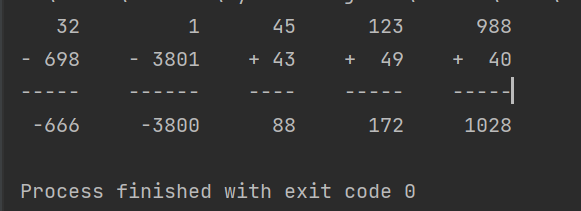# What is the difference between Python 3.8 on Replit and 3.10 with PyCharm

I am currently working on my Scientific Computing with Python degree. I wrote my code with in PyCharm and used Python 3.10. For Testing i just print() and then Checked my results.
While printing the result gave me the wanted results but the tests in replit gave me an error. My question is for the Arithmetic Formatter Challenge.

In Replit i get this error:
arguments = [[‘3 + 855’, ‘988 + 40’], True]
expected_output = ’ 3 988\n+ 855 + 40\n----- -----\n 858 1028’
fail_message = ‘Expected solutions to be correctly displayed in output when calling “arithmetic_arranger()” with [“3 + 855”, “988 + 40”] and a second argument of `True`.’

``````@pytest.mark.parametrize('arguments,expected_output,fail_message', test_cases)
def test_template(arguments, expected_output, fail_message):
``````
``````  actual = arithmetic_arranger(*arguments)
``````

E TypeError: arithmetic_arranger() takes 1 positional argument but 2 were given

test_module.py:76: TypeError

while in PyCharm I get this:I used this:

``````try:
if problems == True:
getSolution = problems
solutions = ""
problems = problems
except:
getSolution = False
``````

to get the True argument out of the 2D.

I hope you can help me with my problem.

greetings from Germany,
Lennart

The optional `True` argument is not part of the `problems` list. It’s a separate argument.

You can run test module in PyCharm as well, you’d get similar error then.

1 Like